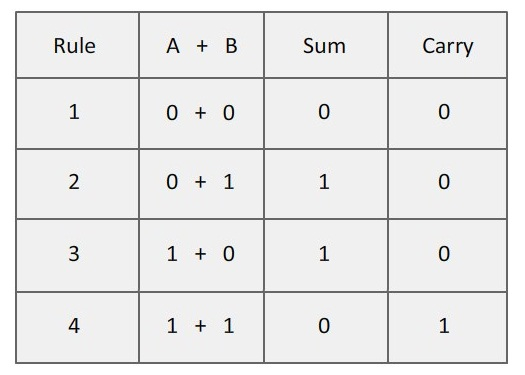Use our binary addition calculator, a free online conversion tool to add two binary values. A Binary number system has only two digits that are 0 and 1. The base of binary number system is 2, because it has only two digits.

#### How to Perform Binary Addition

Despite the fact that most engineers use hex data representation, it has already been shown that logic gates operate on strings of bits that compose each unit of data. Binary arithmetic is performed according to the same rules as decimal arithmetic. When adding two numbers, each column of digits is added in sequence from right to left and, if the sum of any column is greater than the value of the highest digit, a carry is added to the next column. In binary, the largest digit is 1, so any sum greater than 1 will result in a carry.The rules for binary addition are:

0 + 0 = 0 => Sum = 0, carry = 0

0 + 1 = 0 => Sum = 1, carry = 0

1 + 0 = 0 => Sum = 1, carry = 0

1 + 1 = 10 => Sum = 0, carry = 1

When an input carry = 1 due to a previous result, the rules are:

1 + 0 + 0 = 01 => Sum = 1, carry = 0

1 + 0 + 1 = 10 => Sum = 0, carry = 1

1 + 1 + 0 = 10 => Sum = 0, carry = 1

1 + 1 + 1 = 11 => Sum = 1, carry = 1

### Spreading Knowledge Across the World

USA - United States of America  Canada  United Kingdom  Australia  New Zealand  South America  Brazil  Portugal  Netherland  South Africa  Ethiopia  Zambia  Singapore  Malaysia  India  China  UAE - Saudi Arabia  Qatar  Oman  Kuwait  Bahrain  Dubai  Israil  England  Scotland  Norway  Ireland  Denmark  France  Spain  Poland  and  many more....